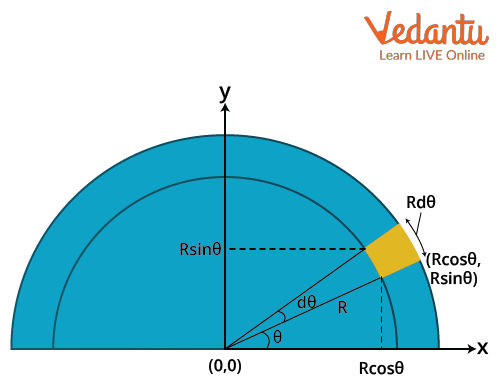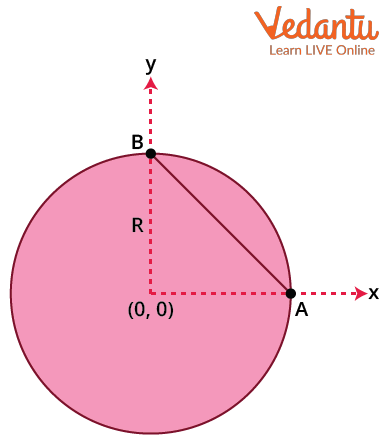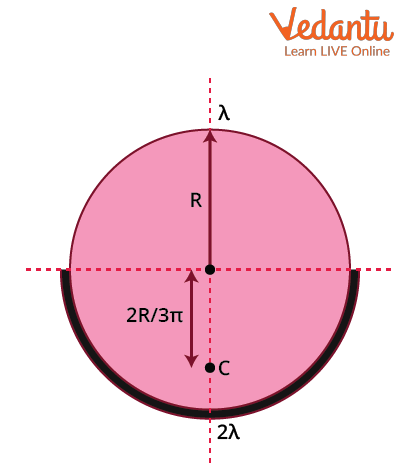Courses
Courses for Kids
Free study material
Free LIVE classes
More

# Semicircular Ring - Centre of Mass and Its Application for JEELIVE
Join Vedantu’s FREE Mastercalss

## Centre of Mass: Brief Introduction

The centre of mass of the system of particles is defined as the point where the mass of the whole body is concentrated for the translatory motion. The motion of this point of the centre of mass can be seen as if the mass of the whole body (i.e., the sum of all the particles present in the body) is concentrated at the centre of mass and all the external forces were applied to this point. In this article, we will be discussing the centre of mass of a semicircular ring and the motion of the centre of mass and will also take a look at the applications of the centre of mass.

## System of Particles

A system can be defined as the collection of a large number of particles that interact with each other. As we know, any real body we encounter in our daily life has a finite size with many particles combined together, called the extended body. The extended body problems can be solved by considering them as rigid bodies. Ideally, a rigid body contains an unchanging and perfectly definite shape, and the distances between the particles remain the same. However, in the real world, deformations do occur for the rigid body and are considered negligible.

The rigid body can have different kinds of motion.

• Translational motion, where all particles of the body have the same velocity at any instant of time.

• Rotational motion, where every particle of the body moves in a circle that lies in a plane perpendicular to the axis and has its centre on the axis.

• The motion of a rigid body which is not pivoted or fixed in some way is either pure translational or a combination of translational and rotational.

• The motion of a rigid body which is pivoted or fixed in some way is rotational.

Example: a ceiling fan rotating about a fixed axis or an oscillating fan moving about the axis.

### Centre of Mass of the System of Particles

The centre of mass for a diverse or discontinuous system at (X,Y,Z) coordinates can be defined as

$X=\dfrac{\sum m_{i} x_{i}}{M}, Y=\dfrac{\sum m_{i} y_{i}}{M}, Z=\dfrac{\sum m_{i} z_{i}}{M}$

Or in general, it can be defined as

$R_{C M}=\dfrac{\sum m_{i} r_{i}}{M}$........(1)

where RCM is the position vector of the centre of mass and ri and mi are the position vector and mass of the ith particle, and M is the total mass of the body.

If the number of particles are more and the system has continuous distribution, the summation is replaced by integrals. The centre of mass RCM with the mass element dm at the position r is given by

$R_{C M}=\dfrac{\int r d m}{M}$............(2)

## COM of Semicircular RingSemicircular ring

Consider the origin and axis of the semicircular ring as shown in the figure to find the COM of the semicircular ring. The ring with the radius R and the angle $\theta$ made by the line joining the origin and the point having coordinates $(R\cos{\theta},~R\sin{\theta})$ on the ring. Consider the infinitely small strip making an angle $d\theta$ having mass dm. As the ring is uniform, the linear mass density is given below

$\lambda=\dfrac{\mathrm{d} m}{\mathrm{~d} x}=\dfrac{M}{\pi R}$  ……..(3)

where M is mass of the ring and 𝜋R is the length of the ring (circumference of a semicircle).

The centre of mass of the ring lies in the geometric centre and if it is cut into two halves horizontally, then the centre of mass of the ring shifts upwards and lies on the y axis. Therefore, the COM of a semicircular ring lies only on the y axis by symmetry.

This can be proved as below. Consider the centre of mass YCM in the y axis with continuous mass distribution

$Y_{C M}=\dfrac{\int y \mathrm{~d} m}{\int d m}$

From diagram $y=Rsin{\theta}$ and $d m=\lambda \mathrm{d} x$ from equation (3)

$\mathrm{d} x=R \mathrm{~d} \theta$ from the infinitesimal strip.

$Y_{C M}=\dfrac{\int R \sin \theta \lambda \mathrm{d} x}{M}=\dfrac{\int R \sin \theta \dfrac{M}{\pi R} \mathrm{~d} x}{M}=\dfrac{1}{\pi} \int \sin \theta R \mathrm{~d} \theta=\dfrac{R}{\pi} \int \sin \theta \mathrm{d} \theta$

The limits are from 0 to $\pi$

$Y_{C M}=\dfrac{R}{\pi} \int_{0}^{\pi} \sin \theta d \theta=\dfrac{R}{\pi}[-\cos \theta]_{0}^{\pi}=\dfrac{R}{\pi}[-\cos \pi+\cos 0]=\dfrac{R}{\pi}[1+1]=\dfrac{2 R}{\pi}$

$Y_{C M}=\dfrac{2 R}{\pi}$...............(4)

Hence, the COM of the semicircular ring.

Similarly for the x axis,

$X_{C M}=\dfrac{\int x \mathrm{~d} m}{\int d m}$

From diagram $x=R\cos{\theta}$ and $d m=\lambda \mathrm{d} x$ from equation (1) $\mathrm{d} x=R \mathrm{~d} \theta$ from the infinitesimal strip.

$X_{C M}=\dfrac{\int R \cos \theta \lambda \mathrm{d} x}{M}=\dfrac{\int R \cos \theta \dfrac{M}{\pi R} \mathrm{~d} x}{M}=\dfrac{1}{\pi} \int \cos \theta R \mathrm{~d} \theta=\dfrac{R}{\pi} \int \cos \theta \mathrm{d} \theta$

The limits are from 0 to $\pi$

$X_{C M}=\dfrac{R}{\pi} \int_{0}^{\pi} \cos \theta d \theta=\dfrac{R}{\pi}[\sin \theta]_{0}^{\pi}=\dfrac{R}{\pi}[\sin \pi-\sin 0]=\dfrac{R}{\pi}=0$

$X_{C M}=0$..........(5)

## Motion of Centre of Mass

As the definition of centre of mass is known, the physical importance for the system of particles can be discussed for n number of particles. From equation (1), it can be written as

$M R=\sum m_{i} r_{i}$

By differentiating both the sides with respect to time,

\begin{align} &M \dfrac{d R}{d t}=m_{i} \sum \dfrac{d r_{i}}{d t} \\ &M \dfrac{d V}{d t}=m_{i} \sum \dfrac{d v_{i}}{d t} \end{align}

From Newton's second law,

Or $M A=m_{i} \sum a_{i}=\sum F_{i}=F_{1}+F_{2}+\ldots+F_{n}$

Each particle experiences both internal and external forces. From Newton's third law, we know that the internal forces occur in equal and opposite pairs, and the sum of forces cancels each other out. Therefore, to determine the motion of the centre of mass of the system, only the external forces are enough.

## Application of Centre of Mass

• To determine the maximum angle required before an object trips or falls.

• If an object is thrown in air, then the path to be traced by it can be predicted.

• It is useful to know the static positions and different movements of the human body during locomotion.

## Solved Examples on Centre of Mass of Semicircular Ring

Example 1: What is the centre of mass of an object consisting of a uniform ring of radius R and a uniform chord AB?Diagram for example 1

Solution:

Centre of mass of the ring is at the centre (0,0).

COM of the uniform rod is at the mid point $\left(\dfrac{R}{2}, \dfrac{R}{2}\right)$

Mass of the ring and rod is m1 and m2, respectively.

Centre of mass of ring in x coordinate is

$X_{C M}=\dfrac{m_{1} \times 0+\left(m_{2} \times \dfrac{R}{2}\right)}{m_{1}+m_{2}}=\dfrac{m_{2}}{m_{1}+m_{2}} \times \dfrac{R}{2}$

And centre of mass of the ring in y coordinate is

$Y_{C M}=\dfrac{m_{1} \times 0+\left(m_{2} \times \dfrac{R}{2}\right)}{m_{1}+m_{2}}=\dfrac{m_{2}}{m_{1}+m_{2}} \times \dfrac{R}{2}$

So, the centre of mass of the ring is $\left(\dfrac{m_{2}}{\left(m_{1}+m_{2}\right)} \dfrac{R}{2}, \dfrac{m_{2}}{\left(m_{1}+m_{2}\right)} \dfrac{R}{2}\right)$.

Example 2: Find the velocity of the centre of mass of two blocks of masses 5 kg and 4 kg con­nected by a spring of negligible mass and placed on a frictionless horizontal surface if the heavier block is given an impulse of velocity 7 $\dfrac{m}{s}$ in the direction of the lighter block.

Solution:

From the equation of motion of the centre of mass, the velocity of the COM can be calculated.

Given mass of the first block as 5 kg and mass of second block 4 kg.

The impulse velocity is 7 $\dfrac{m}{s}$.

By applying the given data, velocity of COM vCM  is

\begin{align} &v_{C M}=\dfrac{m_{1} v_{1}+m_{2} v_{2}}{m_{1}+m_{2}} \\ &v_{C M}=\dfrac{(5 \times 7)+(4 \times 0)}{5+4} \\ &v_{C M}=\dfrac{35}{9} \\ &v_{C M}=3.8 ~\dfrac{\mathrm{m} }{ \mathrm{s} }\end{align}

Hence, the velocity of the centre of mass is 3.8$~\dfrac{\mathrm{m} }{ \mathrm{s} }$.

Example 3: What is the distance of the centre of  mass of the complete ring from its geometrical centre if two semicircular rings of linear mass densities $\lambda$ and $\dfrac{\lambda}{2}$ of radius R are joined to form a complete ring?Diagram for example 3

Solution:

To find the distance of the centre of mass from the geometric centre or the origin.

COM of first ring with linear mass density $\lambda$ is $\left(0, \dfrac{2R}{\pi}\right)$

COM of second ring with linear mass density $\dfrac{\lambda}{2}$  is $\left(0, \dfrac{2R}{\pi}\right)$

COM of the complete ring

$R_{C M}=\dfrac{\left(\lambda \pi \mathrm{R} \times \dfrac{2 \mathrm{R}}{\pi}\right)+\left(\dfrac{\lambda}{2} \times \pi \mathrm{R}\right)\left(\dfrac{-2 \mathrm{R}}{\pi}\right)}{\lambda \pi \mathrm{R}+\dfrac{\lambda}{2} \times \pi \mathrm{R}}=\dfrac{\lambda \mathrm{R}^{2}}{\dfrac{2 \lambda \pi \mathrm{R}+\lambda \pi \mathrm{R}}{2}}=\dfrac{2 R}{3 \pi}$

The distance of the centre of mass from its geometrical centre is 2R/3π.

## Conclusion

The centre of mass of a system of particles is the point whose position vector is $R=\dfrac{\sum m_{i} r_{i}}{M}$ where mi is the mass and ri is the position of the ith particle. The COM of the semicircular ring in the y axis due to symmetry is therefore found to be $Y_{C M}=\dfrac{2 R}{\pi}$. The centre of mass of the ring or any hollow symmetric body can be found at the centre of the body. The motion of the centre of mass of a system can be found only by external forces on the body and does not require knowledge of the internal energy of the system.

Last updated date: 22nd Sep 2023
Total views: 132k
Views today: 2.32k

## FAQs on Semicircular Ring - Centre of Mass and Its Application for JEE

1. Is centroid and centre of mass the same? What is the relationship between the centre of mass and the centre of gravity?

The centroid is the geometric centre of a system or body while the centre of mass is the mean position of the mass of the system. The centre of mass and the centre of gravity are two different terms, but they are often used synonymously. The centre of gravity is the point where gravity or the weight of the system appears to act. It is the sum of all the forces of gravity acting on all the particles in the system. The centre of mass and centre of gravity are the same when the gravitational field is nearly or equally uniform across the body.

2. Why is the centre of mass important?

It is important to locate the centre of mass as it helps to analyse the dynamics of the system based on the motion of the centre of mass. The position of mass from the axis, the mass and the axis of the system affect the centre of mass. In real-life applications, it is a reference point to calculate the mechanics involved in the mass distribution in space, like the linear and angular momentum of planetary bodies and rigid body dynamics.

3. What is the importance of the centre of the mass topic in the JEE exam?

The weightage for this topic is 6.6% and nearly two questions can be asked from this topic in JEE. The questions related to solving the distance of the centre of mass with different bodies as explained and the motion of the centre of mass with varying velocities are asked. The formula for the centre of mass and a few important results like COM of symmetric bodies and asymmetric bodies like semicircular rings need to be remembered.